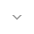belongs to Maven artifact com.android.support:transition:28.0.0-alpha1

# ArcMotion

``` public class ArcMotion ```
``` ``` ``` extends PathMotion ``` ``` ```

 java.lang.Object ↳ android.support.transition.PathMotion ↳ android.support.transition.ArcMotion

A PathMotion that generates a curved path along an arc on an imaginary circle containing the two points. If the horizontal distance between the points is less than the vertical distance, then the circle's center point will be horizontally aligned with the end point. If the vertical distance is less than the horizontal distance then the circle's center point will be vertically aligned with the end point.

When the two points are near horizontal or vertical, the curve of the motion will be small as the center of the circle will be far from both points. To force curvature of the path, `setMinimumHorizontalAngle(float)` and `setMinimumVerticalAngle(float)` may be used to set the minimum angle of the arc between two points.

This may be used in XML as an element inside a transition.

``````<changeBounds>
<arcMotion android:minimumHorizontalAngle="15"
android:minimumVerticalAngle="0"
android:maximumAngle="90"/>
</changeBounds>```
```

## Summary

### Public constructors

``` ArcMotion() ```
``` ArcMotion(Context context, AttributeSet attrs) ```

### Public methods

``` float``` ``` getMaximumAngle() ```

Returns the maximum arc along the circle between two points.

``` float``` ``` getMinimumHorizontalAngle() ```

Returns the minimum arc along the circle between two points aligned near horizontally.

``` float``` ``` getMinimumVerticalAngle() ```

Returns the minimum arc along the circle between two points aligned near vertically.

``` Path``` ``` getPath(float startX, float startY, float endX, float endY) ```

Provide a Path to interpolate between two points `(startX, startY)` and `(endX, endY)`.

``` void``` ``` setMaximumAngle(float angleInDegrees) ```

Sets the maximum arc along the circle between two points.

``` void``` ``` setMinimumHorizontalAngle(float angleInDegrees) ```

Sets the minimum arc along the circle between two points aligned near horizontally.

``` void``` ``` setMinimumVerticalAngle(float angleInDegrees) ```

Sets the minimum arc along the circle between two points aligned near vertically.

### Inherited methodsFrom class ``` android.support.transition.PathMotion ```From class ``` java.lang.Object ```

## Public constructors

### ArcMotion

`ArcMotion ()`
`ArcMotion (`